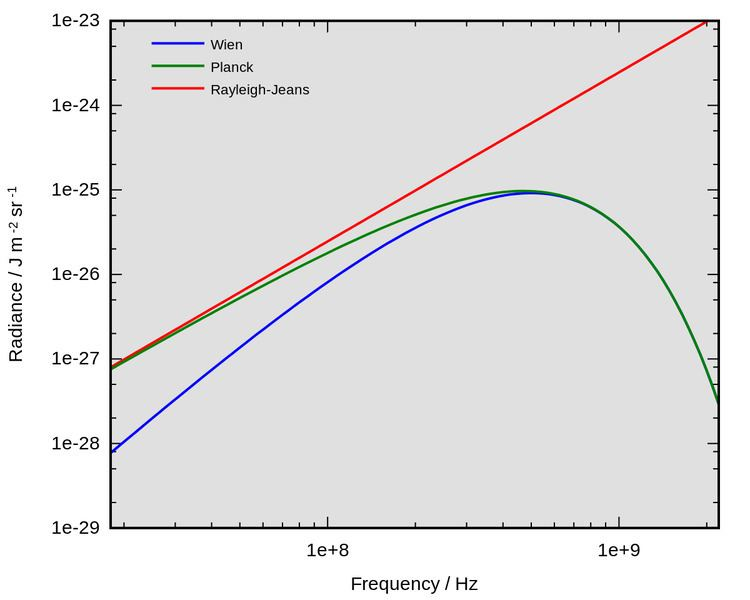# Wien approximation

Updated on
Covid-19Wien's approximation (also sometimes called Wien's law or the Wien distribution law) is a law of physics used to describe the spectrum of thermal radiation (frequently called the blackbody function). This law was first derived by Wilhelm Wien in 1896. The equation does accurately describe the short wavelength (high frequency) spectrum of thermal emission from objects, but it fails to accurately fit the experimental data for long wavelengths (low frequency) emission.

## Details

Wien derived his law from thermodynamic arguments, several years before Planck introduced the quantization of radiation. Details are contained in. The law may be written as

I ( ν , T ) = 2 h ν 3 c 2 e h ν k T

where

• I ( ν , T ) is the amount of energy per unit surface area per unit time per unit solid angle per unit frequency emitted at a frequency ν.
• T is the temperature of the black body.
• h is Planck's constant.
• c is the speed of light.
• k is Boltzmann's constant.
• This equation may also be written as

I ( λ , T ) = 2 h c 2 λ 5 e h c λ k T

where I ( λ , T ) is the amount of energy per unit surface area per unit time per unit solid angle per unit wavelength emitted at a wavelength λ.

The peak value of this curve, as determined by taking the derivative and solving for zero, occurs at a wavelength λmax and frequency νmax of:

λ m a x T   =   0.288   c m K ν m a x   =   5.88 × 10 10 T

in cgs units.

## Relation of Planck's law to the Wien approximation

The Wien approximation was originally proposed as a description of the complete spectrum of thermal radiation, although it failed to accurately describe long wavelength (low frequency) emission. However, it was soon superseded by Planck's law, developed by Max Planck. Unlike the Wien approximation, Planck's law accurately describes the complete spectrum of thermal radiation. Planck's law may be given as

I ( ν , T ) = 2 h ν 3 c 2 1 e h ν k T 1

The Wien approximation may be derived from Planck's law by assuming h ν k T . When this is true, then

1 e h ν k T 1 e h ν k T

and so Planck's law approximately equals the Wien approximation at high frequencies.

## Other approximations of thermal radiation

The Rayleigh–Jeans law developed by Lord Rayleigh may be used to accurately describe the long wavelength spectrum of thermal radiation but fails to describe the short wavelength spectrum of thermal emission.

## References

Similar Topics
The Other Side (2006 film)
Robert ten Brink
Jaromír Musil
Topics